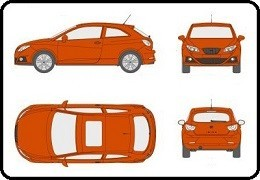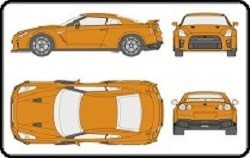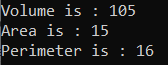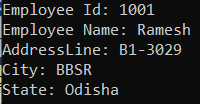# IsA and HasA Relationship in C#

## IsA and HasA Relationship in C# with Examples

In this article, I am going to discuss IsA and HasA Relationships in C# with Examples. Please read our Inheritance in C# article before proceeding to this article. It is very important to understand the concept of IS-A and HAS-A relationship between classes. Many students, many developers, especially beginners and freshers get confused between the IS-A and HAS-A relationship and because of this, they are making the relationship between classes in the wrong order. So, today, I will explain what is exactly IS-A and HAS-A relationships, what is the differences between them, and when to use and how to use IS-A and HAS-A relationships in real-time application. Here, I am going to show the examples using the C# language but this is also applicable to any other object-oriented programming language Java, C++, etc.

##### IsA and HasA Relationship in C# with Examples:

Let us understand IS-A and HAS-A Relationship in C# with one example. Please have a look at the below example.

class Rectangle{
Some Data Members
Some Member Functions
}

This is a class called Rectangle. Let us assume data we have some data members and member functions there inside this class. Next, we have a class called Cuboid which is inheriting from the above Rectangle class as follows.

class Cuboid : Rectangle{
Some Data Members
Some Member Functions
}

This is the Cuboid class which is inherited from the Rectangle class. Also, assume that this class also has some data members and member functions inside it. Now let us write one more class as follows.

class Table{
Rectangle top;
int legs;
}

This is the Table class which is not inherited from any class. This class has two data members. One of the data members is of class Rectangle and the other is of integer type variable i.e. top and legs.

So first we have created a class called Rectangle. Then we created another class called Cuboid which is inherited from the Rectangle class and then we created another class called Table. Inside the Table class, we have created one variable of the type Rectangle as well as another variable of integer type.

The class Cuboid is inherited from the Rectangle class. So, can we say that a Cuboid IA A Rectangle? Yes. So, the relationship between the Rectangle class and Cuboid class is the Is A relationship. So, the point that you need to remember is whenever we are making the Inheritance relationship or Parent-Child relationship, then we can say that relationship as IS-A relationship in object-oriented programming languages.

Next, our Table class, it is having a top variable that is of the type Rectangular. That means the Table class is having an object of the Rectangle class. So, can we say that the Table class HAS A Rectangle? Yes, the Table class Has a Rectangle. So, the relationship between the Table class and the Rectangle class is the Has A relationship.

So, we can use our class in two ways that are ‘Is A’ and ‘Has A’. This is common in object-oriented programming languages just not for C#, it is available in C++, Java, and other Object-Oriented Programming Language.

So, a class in C# can be used in two ways. One way is by using the Inheritance relationship i.e. one class is inheriting from that class. The second approach is that you can create an object of that class and use it. So, there are two ways of using one class. Either you can create the object and use it or you can inherit it from that class.

##### Real-time Example to Understand Is-A and Has-A Relationship:

Let us understand IS-A and HAS-A relationships with one Real-time Example. Let us take an example. Please have a look at the below image.Let us assume that the above image shows the design of my car. Now I can manufacture any number of cars from the above design and I can sell them on the market. Let us assume we have manufactured the following cars from the above design.Manufacturing the Cars means, these are objects. Now I can sell my design (not the manufactured cars or objects, only the design) to your company and you can manufacture your car with some changes in the above design which I sell to you.##### So, what are the uses of design class?

What is the use of the design class means in how many ways we can use the design class? We can use the design class in two ways. Either we share it with the derived classes or create an object. If a class is inheriting from some other class, then it is having an ‘IS A’ relationship with that class or if a class is having an object of some other class, then it is having a ‘HAS A’ relationship with that class. So, Table has a Rectangle, and a Cuboid is a Rectangle.

Next, important thing is that a class can have different types of members i.e. we have six types of members such as public, private, protected, internal, protected internal, and private protected. But, let us assume that we have three types of members in our class such as privateprotected, and public.

Now when you have those three types of members then which members are accessible inside the class, which members are accessible in the class which is having ‘IS A’ relationship, and which members are accessible in the class which is having ‘HAS A’ relationship? So, it means what is accessible inside the class and what is accessible in derived classes, and what is accessible upon an object. We already discussed this in our Access Specifiers article. All the members are accessible within the class, public and protected members are accessible in the derived classes (IS-A relationship) and public members are only accessible from non-derived classes (HAS-A relationship).

##### Example to Understand IS-A Relationship in C#

Please have a look at the below example. In the below example, the relation between Rectangle and Cuboid is IS-A relationship, so we can say that Cuboid IS-A Rectangle.

```using System;
{
public class Program
{
static void Main()
{
Cuboid cuboid = new Cuboid(3, 5, 7);
Console.WriteLine(\$"Volume is : {cuboid.Volume()}");
Console.WriteLine(\$"Area is : {cuboid.Area()}");
Console.WriteLine(\$"Perimeter is : {cuboid.Perimeter()}");

}
}
class Rectangle
{
//Data Members
public int Length;

//Member Functions
public int Area()
{
}
public int Perimeter()
{
return 2 * (Length + Breadth);
}
}

//Establishing Parent-Child Relationship
//IS-A Relationship i.e. Cuboid IS-A Rectangle
class Cuboid : Rectangle
{
public int Height;
public Cuboid(int l, int b, int h)
{
Length = l;
Height = h;
}
public int Volume()
{
return Length * Breadth * Height;
}
}
}
```
###### Output:##### Example to Understand HAS-A Relationship in C#

Please have a look at the below example. In the below example, within the Employee class, we have created one variable of Address type which is nothing but a HAS-A relationship between Employee and Address. So, we can say that Employee HAS-A Address.

```using System;
{
public class Program
{
static void Main()
{
Employee employee = new Employee(1001, "Ramesh", address);
employee.Display();
}
}
{
{
City = city;
State = state;
}
}
class Employee
{
//Establishing Has-A relationship i.e. Employee HAS-A Address
public int Id;
public string Name;
{
Id = id;
Name = name;
}
public void Display()
{
Console.WriteLine(\$"Employee Id: {Id}");
Console.WriteLine(\$"Employee Name: {Name}");
}
}
}
```
###### Output:In the above example, if we inherit the Employee Class from the Address class, then it does not make any sense. Because if we inherit then it will establish an IS-A relationship between Employee and Address classes and it does not make any sense to say Employee IS-A Address rather if we implement HAS-A relationship, then it makes sense to say Employee HAS-A Address.

##### How to Decide What to Implement between IS-A and HAS-A?

Simply, ask the question yourself. For example, If I ask you the questions which statement gives you more sense from the below two statements?

Then definitely, you will tell Employee HAS-A Address gives more sense than Employee IS-A Address. Now, again If I ask you, which statement gives you a better sense of the below two statements?

BMW IS-A Car
BMW HAS-A Car

Note: In C#, the IS-A relationship is implemented using Inheritance and the HAS-A relationship is implemented using Composition i.e. declaring a variable. So, whenever we declare a variable of one class inside another class, we called it a Composition or you can say HAS-A relationship.

1.2.